## Friday, July 7, 2017

### Slides for Evolution 2017: A phytools plotting demo

The following is a demo of the phytools functions & methods I used to create the graphics for my Evolution 2017 meeting talk entitled “Some additional new methods for plotting phylogenies & comparative data.” The final slides for this talk are available here.

``````library(phytools)
packageVersion("phytools")
``````
``````##  '0.6.18'
``````
``````## -- Read some data from file:

## -- These methods simply plot the data at the tips of the tree. There also
## -- exists a number of other comparable functions.

dotTree(tree,x,length=10,ftype="i")
``````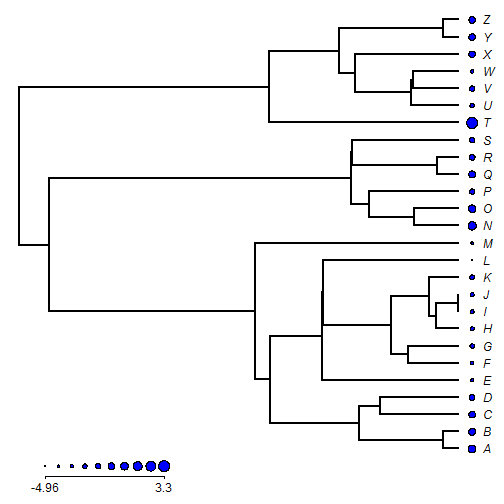``````plotTree.barplot(tree,x)
``````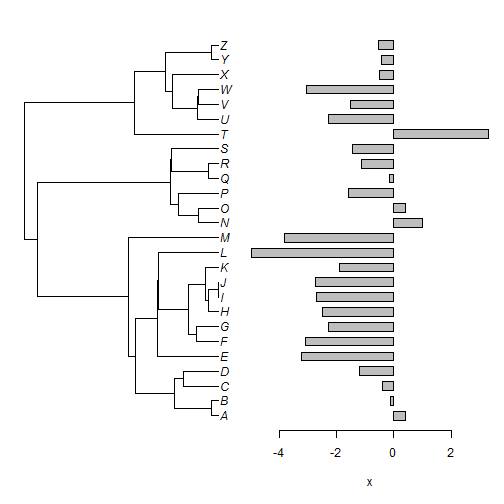``````plotTree.barplot(tree,x,args.barplot=list(col=sapply(x,
function(x) if(x>=0) "blue" else "red"),
xlim=c(-4,4)))
``````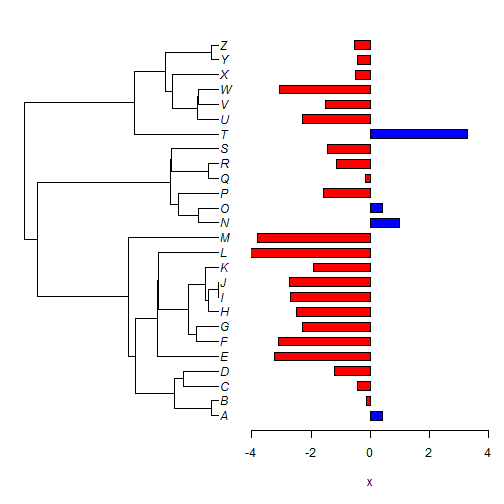``````## -- We can also plot the data at the tips of the tree for multiple traits
## -- simultaneously. For instance:

dotTree(tree,X,standardize=TRUE,length=10)
``````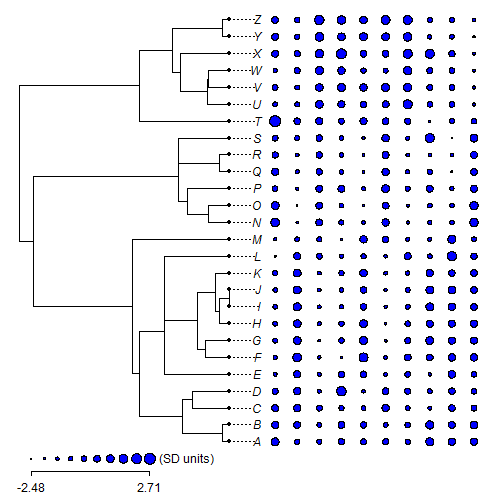``````phylo.heatmap(tree,X,standardize=TRUE)
``````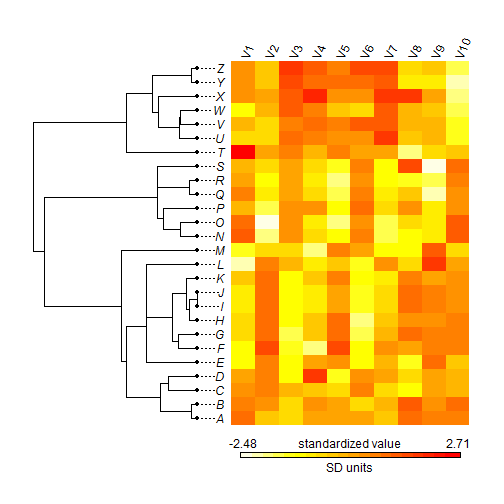``````## -- Aside from plotting the data at the tips, we can also visualize its
## -- reconstructed evolution. A simple method for doing this is called a
## -- 'traitgram' in which the phylogeny is projected into phenotype space
## -- as follows:

``````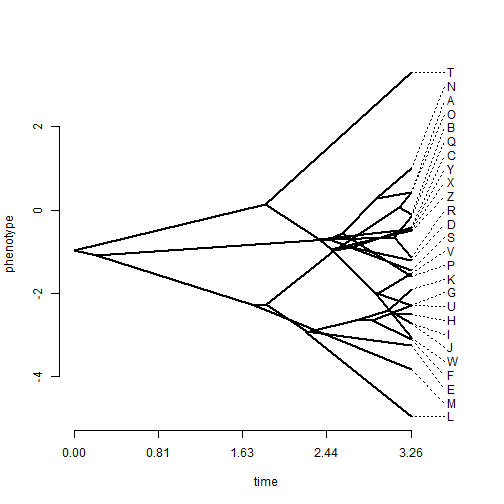``````## -- A variant of this function exists where the idea is to visualize the
## -- uncertainty around the reconstructed values at internal nodes, as
## -- follows:

``````
``````## Computing density traitgram...
``````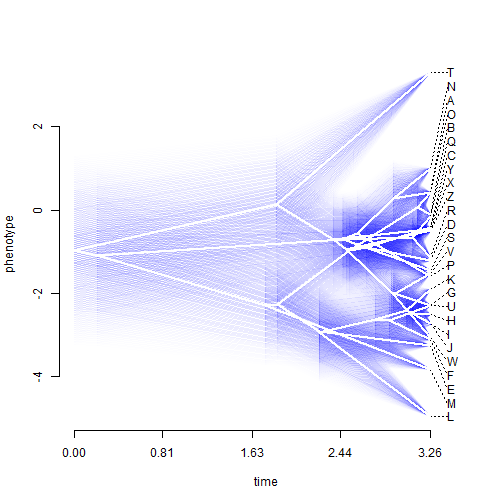``````## -- Another method in phytools projects reconstructed states onto internal
## -- edges & nodes of the tree using a color gradient. I will also demonstrate
## -- a helper function called errorbar.contMap which computes & adds error
## -- bars to the tree using the same color gradient as was employed in the
## -- plot.

obj<-contMap(tree,x,plot=FALSE)
plot(obj,lwd=7,xlim=c(-0.2,3.6))
errorbar.contMap(obj)
``````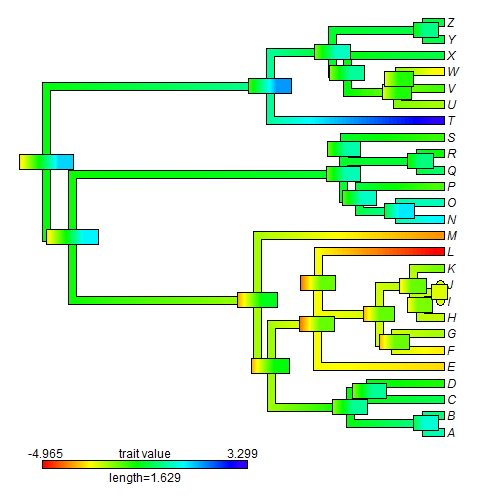``````## -- This method creates a special object class that can then be replotted
## -- without needing to repeat all the computation. We can also replot this
## -- object using different parameters. For example, in the following script
## -- I will invert & then gray-scale the color gradient of our original plot
## -- & then replot these two trees in a facing manner.

obj
``````
``````## Object of class "contMap" containing:
##
## (1) A phylogenetic tree with 26 tips and 25 internal nodes.
##
## (2) A mapped continuous trait on the range (-4.964627, 3.299418).
``````
``````obj<-setMap(obj,invert=TRUE)
grey<-setMap(obj,c("white","black"))
par(mfrow=c(1,2))
plot(obj,lwd=7,ftype="off",xlim=c(-0.2,3.6),legend=2)
plot(grey,lwd=7,direction="leftwards",ftype="off",xlim=c(-0.2,3.6),
legend=2)
``````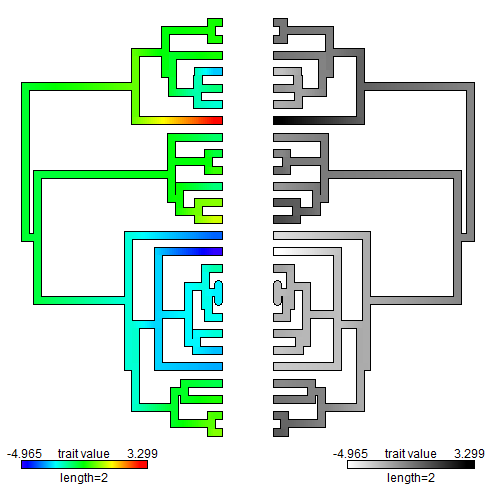``````## -- Now let's try the same thing but with some empirical data:

anole.tree
``````
``````##
## Phylogenetic tree with 100 tips and 99 internal nodes.
##
## Tip labels:
##  ahli, allogus, rubribarbus, imias, sagrei, bremeri, ...
##
## Rooted; includes branch lengths.
``````
``````svl<-read.csv("svl.csv",header=TRUE,row.names=1)
svl<-setNames(svl[,1],rownames(svl))
obj<-contMap(anole.tree,svl,plot=FALSE)
obj<-setMap(obj,invert=TRUE)
plot(obj,fsize=c(0.4,1),outline=FALSE,lwd=c(3,7),leg.txt="log(SVL)")
``````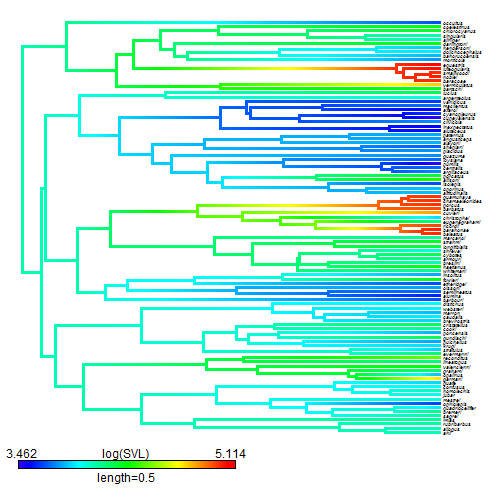``````## -- This plotting method is fairly flexible, as we've seen. We can also
## -- plot our tree in a circular or "fan" style. In the following demo,
## -- I will also show how to add raster images of some of the taxa to
## -- our plot, which is kind of cool.

plot(obj,type="fan",outline=FALSE,fsize=c(0.6,1),legend=1,
lwd=c(4,8),xlim=c(-1.8,1.8),leg.txt="log(SVL)")
spp<-c("barbatus","porcatus","cristatellus","equestris","sagrei")
library(png)
w<-0.5
for(i in 1:length(spp)){
arrl<-if(spp[i]%in%c("sagrei","porcatus")) 0.24
else if(spp[i]=="barbatus") 0.2 else 0.34
asp<-dim(img)/dim(img)
rasterImage(img,xy\$x0-w/2,xy\$y0-w/2*asp,xy\$x0+w/2,xy\$y0+w/2*asp)
}
``````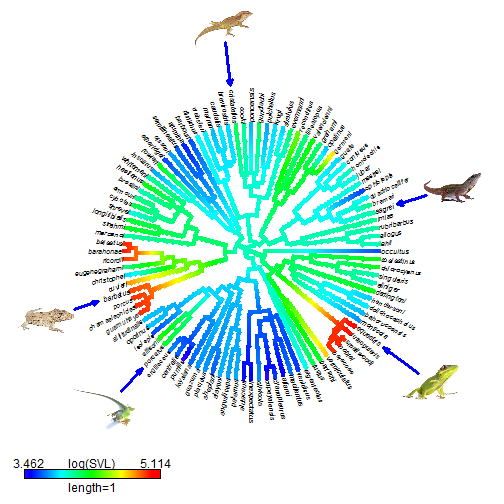``````## -- Another popular visualization method in phytools is something called a
## -- phylomorphospace, which is just a projection of the tree into (normally)
## -- a two-dimensional morphospace. For example:

phylomorphospace(tree,X[,1:2],xlab="trait 1",ylab="trait 2")
``````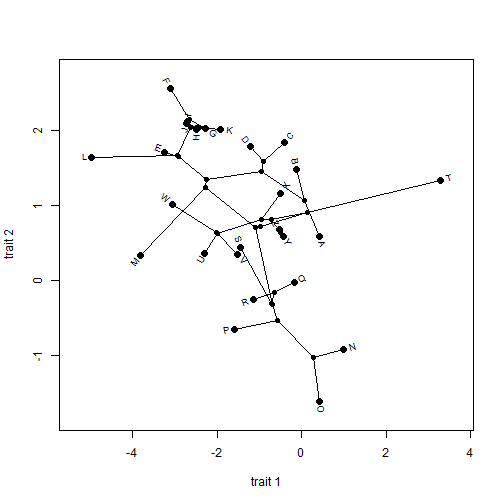``````## -- For more than three continuous trait dimensions phytools also has a method
## -- that I really like, although I've literally never seen it used in
## -- publication. I call this method a 'phylogenetic scatterplot matrix'
## -- because it contains unidimensional contMap plots on the diagonal &
## -- bivariate phylomorphospaces for each pair of traits in off-diagonal
## -- positions. This is what that looks like for five traits:

obj<-fancyTree(tree,type="scattergram",X=X[,1:5])
``````
``````## Computing multidimensional phylogenetic scatterplot matrix...
``````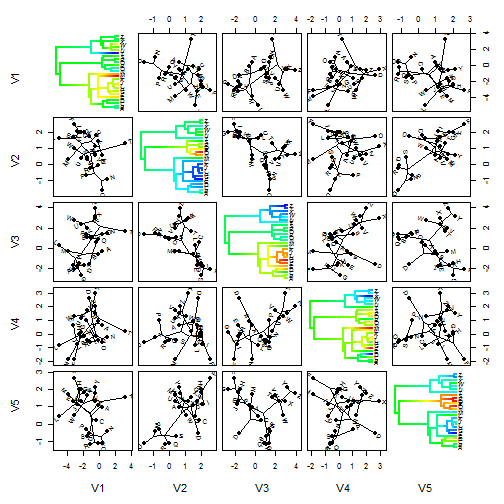``````## -- In addition to these continuous character methods, phytools also has a
## -- number of discrete character visualization tools for plotting discrete
## -- valued traits & trees.

## -- For instance, phytools contains a range of different methods related
## -- to a statistical approach called stochastic character mapping, in which
## -- dicrete character histories are sampled from their posterior probability
## -- distribution. For starters, I will just use a stochastic character map
## -- tree that can be loaded from the package.

data(anoletree)
cols<-setNames(palette()[1:6],mapped.states(anoletree))
plot(anoletree,cols,type="fan",fsize=0.8,lwd=3,ftype="i")
y=0.9*par()\$usr,prompt=FALSE,fsize=0.9)
``````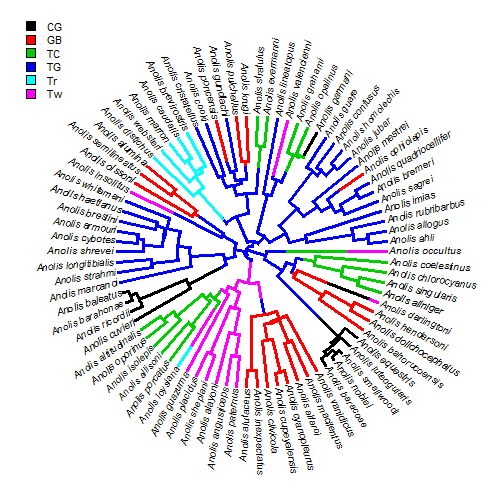``````## -- Normally, we would be working with a set of stochastic character
## -- histories, not just a single such history. Here's an example in which
## -- we first do stochastic mapping, & then we plot the posterior
## -- probabilities that each node is in each state.

ecomorph<-as.factor(getStates(anoletree,"tips"))
trees<-make.simmap(anoletree,ecomorph,model="ER",nsim=100)
``````
``````## make.simmap is sampling character histories conditioned on the transition matrix
##
## Q =
##             CG          GB          TC          TG          Tr          Tw
## CG -0.11570723  0.02314145  0.02314145  0.02314145  0.02314145  0.02314145
## GB  0.02314145 -0.11570723  0.02314145  0.02314145  0.02314145  0.02314145
## TC  0.02314145  0.02314145 -0.11570723  0.02314145  0.02314145  0.02314145
## TG  0.02314145  0.02314145  0.02314145 -0.11570723  0.02314145  0.02314145
## Tr  0.02314145  0.02314145  0.02314145  0.02314145 -0.11570723  0.02314145
## Tw  0.02314145  0.02314145  0.02314145  0.02314145  0.02314145 -0.11570723
## (estimated using likelihood);
## and (mean) root node prior probabilities
## pi =
##        CG        GB        TC        TG        Tr        Tw
## 0.1666667 0.1666667 0.1666667 0.1666667 0.1666667 0.1666667
``````
``````## Done.
``````
``````obj<-summary(trees,plot=FALSE)
plot(obj,colors=cols,type="fan",fsize=0.8,cex=c(0.5,0.3))
y=0.9*par()\$usr,prompt=FALSE,fsize=0.9)
``````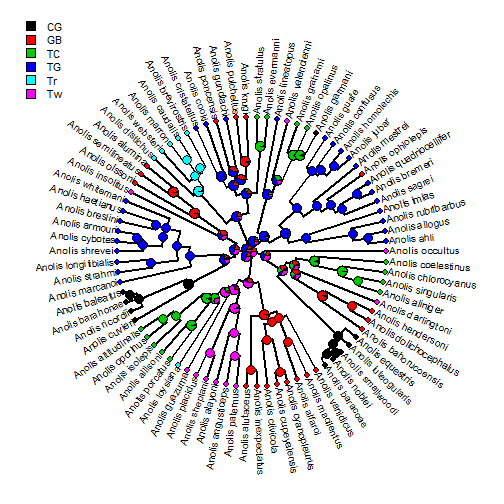``````## -- These are not visualizations for phylogenies, per se, but there are
## -- several other related plotting methods in the phytools that we can
## -- employ here. For instance, we can visualize our fitted discrete
## -- character model:

fit.ARD<-fitMk(anoletree,ecomorph,model="ARD")
plot(fit.ARD,main="fitted ARD model for ecomorph evolution")
``````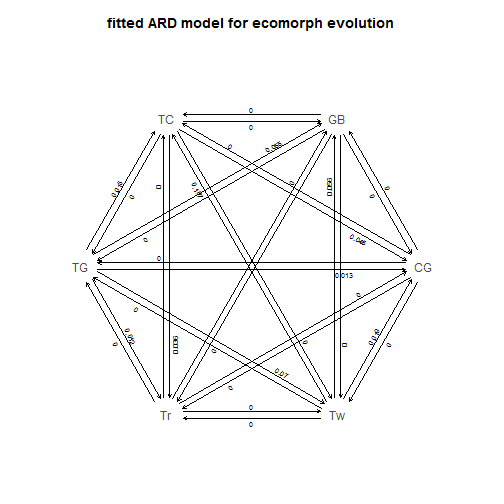``````plot(fit.ARD,show.zeros=FALSE,main="fitted ARD model for ecomorph evolution")
``````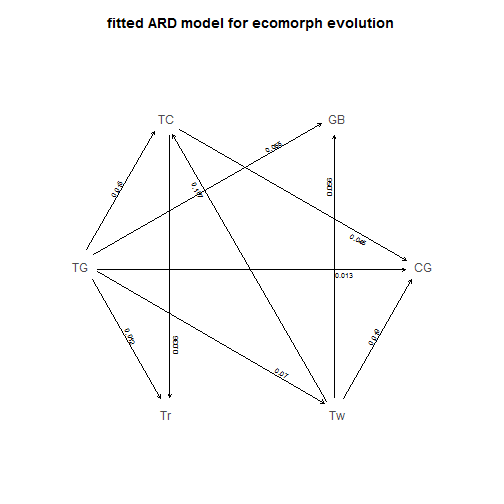``````## -- We can also visualize the posterior density of changes of different types
## -- on the tree. For example:

dd<-density(trees)
plot(dd)
``````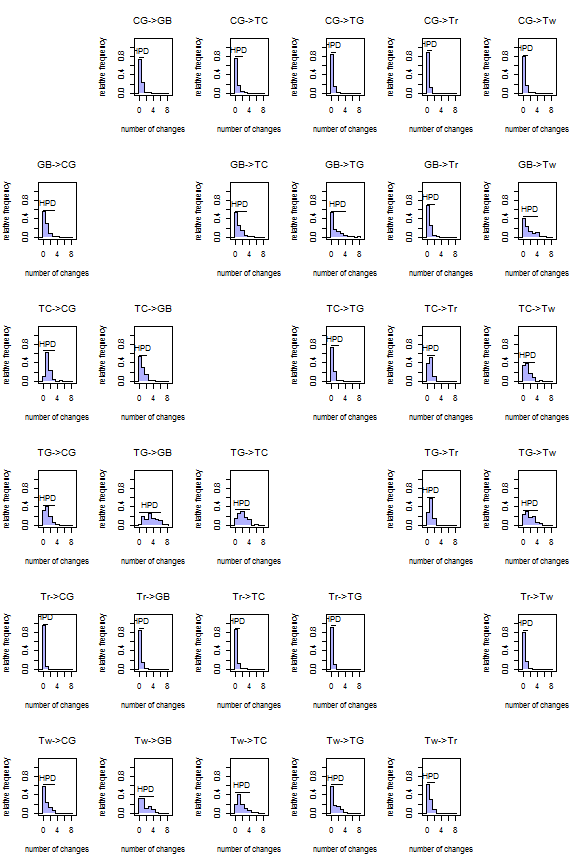``````## -- For binary traits we have more options still. In this example we will
## -- use a different empirical dataset consisting of feeding mode (biting vs.
## -- suction feeding) in elomorph eels. The first plot is just the character
## -- distribution at the tips; whereas the second is the posterior density
## -- from stochastic mapping at the edges & nodes of the tree.

fmode<-as.factor(setNames(eel.data[,1],rownames(eel.data)))
dotTree(eel.tree,fmode,colors=setNames(c("blue","red"),
c("suction","bite")),ftype="i",fsize=0.7)
``````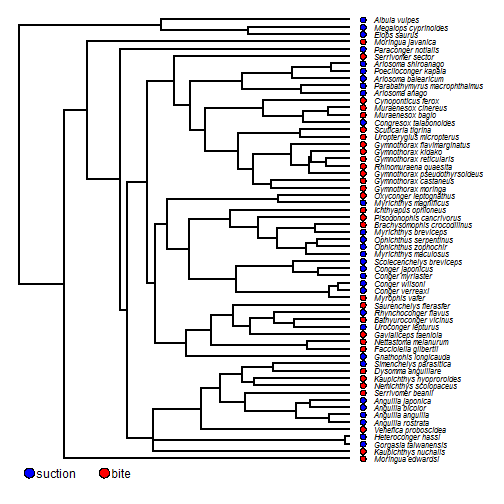``````eel.trees<-make.simmap(eel.tree,fmode,nsim=100)
``````
``````## make.simmap is sampling character histories conditioned on the transition matrix
##
## Q =
##                bite     suction
## bite    -0.01582783  0.01582783
## suction  0.01582783 -0.01582783
## (estimated using likelihood);
## and (mean) root node prior probabilities
## pi =
##    bite suction
##     0.5     0.5
``````
``````## Done.
``````
``````obj<-densityMap(eel.trees,states=c("suction","bite"),plot=FALSE)
``````
``````## sorry - this might take a while; please be patient
``````
``````plot(obj,lwd=4,outline=TRUE,fsize=c(0.7,0.9),legend=50)
``````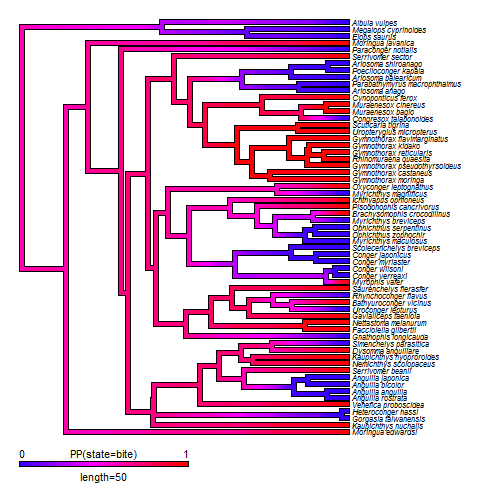``````## -- Or we can show the full posterior sample of changes of each type on the
## -- tree. These are not changes from any single stochastic character history,
## -- but changes in our full posterior sample of such histories.

dotTree(eel.tree,fmode,colors=setNames(c("red","blue"),c("bite","suction")),
fsize=0.7,ftype="i",legend=FALSE)
"suction")),prompt=FALSE,vertical=FALSE,shape="circle")
nulo<-sapply(eel.trees,markChanges,sapply(setNames(c("red","blue"),
c("bite","suction")),make.transparent,0.05))
c("bite","suction"))[2:1],
c("bite->suction","suction->bite")),
make.transparent,0.1),prompt=FALSE,x=50,y=-4,vertical=FALSE)
``````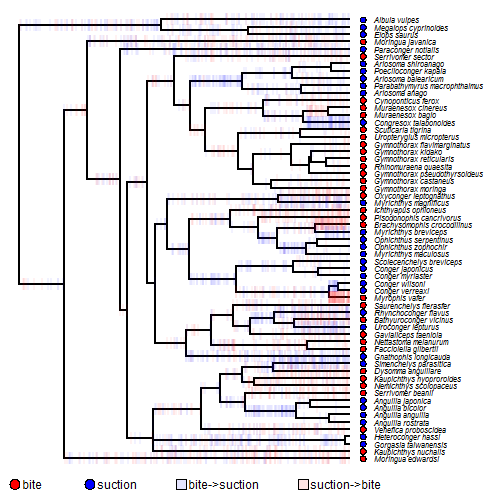``````## -- In addition to these methods for either continuous or discretely
## -- measured character traits, we can easily combine the methods.

## -- For instance, in the following I will overlay the posterior density
## -- from stochastic mapping on a dotTree plot of the continuous trait of
## -- overall body size.

bsize<-setNames(eel.data[,2],rownames(eel.data))
dotTree(eel.tree,bsize,length=10,fsize=0.7,lwd=7,ftype="i")
text(x=28,y=-1.8,"body size (cm)",pos=4)
xlim=get("last_plot.phylo",envir=.PlotPhyloEnv)\$x.lim,
ylim=get("last_plot.phylo",envir=.PlotPhyloEnv)\$y.lim)
prompt=FALSE,x=70,y=-2)
``````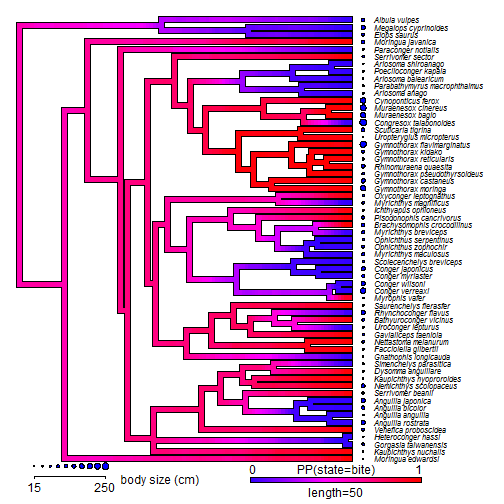``````## -- Alternatively, we could do this as two facing plots - one of densityMap
## -- and the other of contMap styles:

layout(matrix(1:3,1,3),widths=c(0.39,0.22,0.39))
plot(obj,lwd=6,ftype="off",legend=60,outline=TRUE,fsize=c(0,1.2))
plot.new()
plot.window(xlim=c(-0.1,0.1),
ylim=get("last_plot.phylo",envir=.PlotPhyloEnv)\$y.lim)
par(cex=0.6)
text(rep(0,length(obj\$tree\$tip.label)),1:Ntip(obj\$tree),
gsub("_"," ",obj\$tree\$tip.label),font=3)
plot(setMap(contMap(eel.tree,bsize,plot=FALSE),invert=TRUE),lwd=6,outline=TRUE,
direction="leftwards",ftype="off",legend=60,fsize=c(0,1.2),
leg.txt="body size (cm)")
``````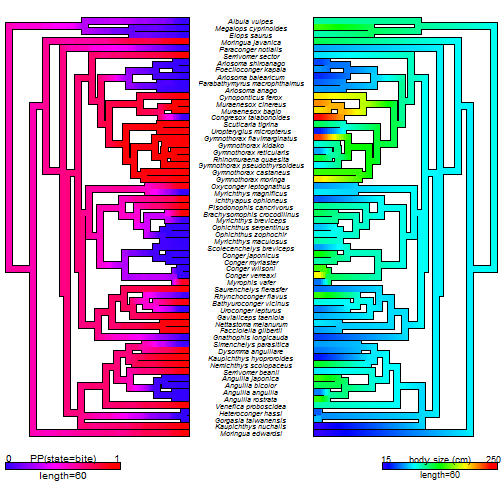``````## -- As another example here is a stochastically mapped discrete character
## -- history overlain on a traitgram plot of body size. At nodes I will add
## -- the posterior probabilties from stochastic mapping.

phenogram(eel.trees[],bsize,lwd=3,colors=
setNames(c("blue","red"),c("suction","bite")),
ftype="i")
``````
``````## Optimizing the positions of the tip labels...
``````
``````add.simmap.legend(colors=setNames(c("blue","red"),c("suction","bite")),
prompt=FALSE,shape="circle",x=0,y=250)
obj<-summary(eel.trees)
nodelabels(pie=obj\$ace,piecol=setNames(c("red","blue"),colnames(obj\$ace)),
cex=0.6)
tiplabels(pie=to.matrix(fmode[eel.tree\$tip.label],colnames(obj\$ace)),
piecol=setNames(c("red","blue"),colnames(obj\$ace)),cex=0.4)
``````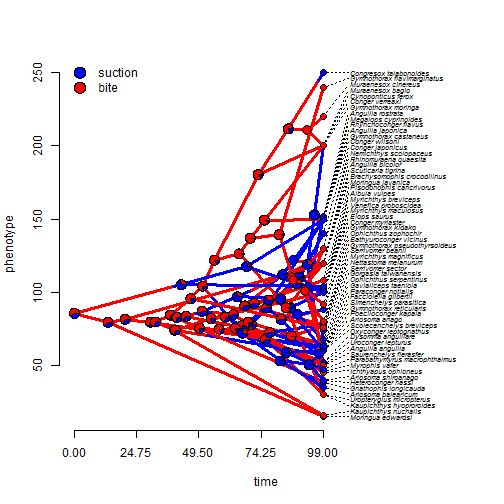``````## -- These are just a few examples. There are many other ways in which
## -- discrete & continuous character methods can be combined
``````
``````## -- I'm now going to demonstrate a handful of other phytools plotting methods.

## -- Firstly, phytools can be used to project a tree onto a geographic map.
## -- This can be done using linking lines from the tips of a square phylogram
## -- or directly.

obj<-phylo.to.map(tree,lat.long,plot=FALSE)
``````
``````## objective: 110
## objective: 110
## objective: 110
## objective: 110
## objective: 110
## objective: 110
## objective: 110
## objective: 110
``````
``````## objective: 100
## objective: 100
``````
``````## objective: 98
## objective: 98
## objective: 98
## objective: 98
## objective: 98
## objective: 98
``````
``````## objective: 96
``````
``````## objective: 92
## objective: 92
``````
``````## objective: 86
## objective: 86
## objective: 86
## objective: 86
## objective: 86
## objective: 86
``````
``````plot(obj,type="phylogram",asp=1.3,mar=c(0.1,0.5,3.1,0.1))
``````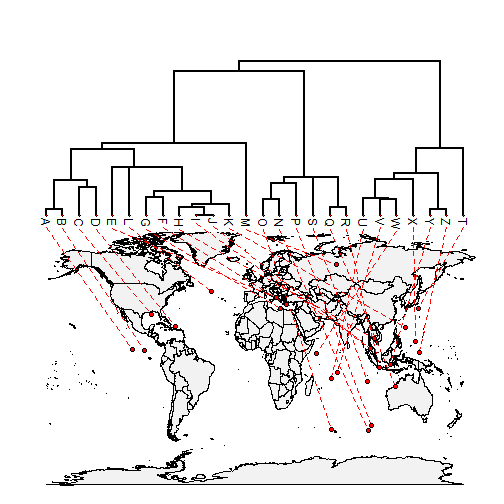``````plot(obj,type="direct",asp=1.3,mar=c(0.1,0.5,3.1,0.1))
``````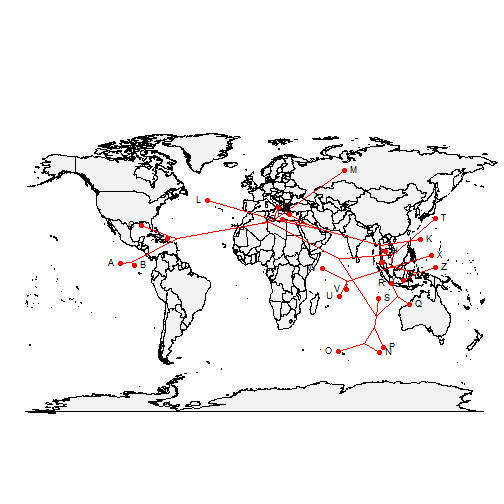``````## -- phytools can be used to create a co-phylogenetic plot in which two
## -- trees are graphed in a facing fashion, with linking lines between
## -- corresponding tips. The following uses data from Lopez-Vaamonde et al.
## -- (2001; MPE).

Pleistodontes<-trees[]
Sycoscapter<-trees[]
obj<-cophylo(Pleistodontes,Sycoscapter,assoc=assoc,
print=TRUE)
``````
``````## Rotating nodes to optimize matching...
``````
``````## objective: 359.577777777778
## objective: 359.577777777778
## objective: 359.577777777778
## objective: 359.577777777778
## objective: 359.577777777778
## objective: 359.577777777778
## objective: 359.577777777778
## objective: 359.577777777778
## objective: 359.577777777778
## objective: 359.577777777778
## objective: 359.577777777778
## objective: 359.577777777778
``````
``````## objective: 357.777777777778
## objective: 357.777777777778
``````
``````## objective: 345.111111111111
## objective: 345.111111111111
## objective: 345.111111111111
``````
``````## objective: 322.777777777778
``````
``````## objective: 201.17728531856
## objective: 201.17728531856
## objective: 201.17728531856
## objective: 201.17728531856
## objective: 201.17728531856
## objective: 201.17728531856
## objective: 201.17728531856
## objective: 201.17728531856
## objective: 201.17728531856
## objective: 201.17728531856
## objective: 201.17728531856
## objective: 201.17728531856
## objective: 201.17728531856
## objective: 201.17728531856
``````
``````## objective: 322.777777777778
## objective: 322.777777777778
## objective: 322.777777777778
## objective: 322.777777777778
## objective: 322.777777777778
## objective: 322.777777777778
## objective: 322.777777777778
## objective: 322.777777777778
## objective: 322.777777777778
## objective: 322.777777777778
## objective: 322.777777777778
## objective: 322.777777777778
## objective: 322.777777777778
## objective: 322.777777777778
## objective: 322.777777777778
## objective: 322.777777777778
## objective: 322.777777777778
## objective: 322.777777777778
``````
``````## objective: 201.17728531856
## objective: 201.17728531856
## objective: 201.17728531856
## objective: 201.17728531856
## objective: 201.17728531856
## objective: 201.17728531856
## objective: 201.17728531856
## objective: 201.17728531856
## objective: 201.17728531856
## objective: 201.17728531856
## objective: 201.17728531856
## objective: 201.17728531856
## objective: 201.17728531856
## objective: 201.17728531856
``````
``````## Done.
``````
``````plot(obj,link.type="curved",link.lwd=3,link.lty="solid",
``````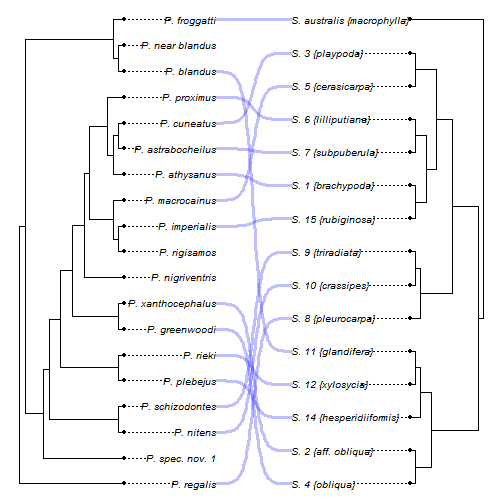``````## -- This method is fairly flexible. For instance, we can use add tip, node,
## -- or edge labels to each tree, or we can us different colors for different
## -- edges.

nodelabels.cophylo(which="left",frame="circle",cex=0.8)
nodelabels.cophylo(which="right",frame="circle",cex=0.8)
``````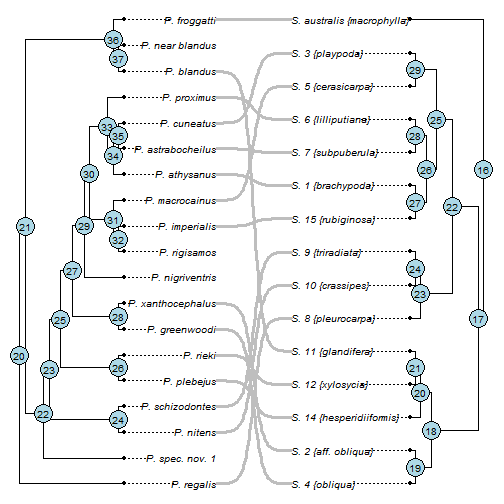``````left<-rep("black",nrow(obj\$trees[]\$edge))
nodes<-getDescendants(obj\$trees[],30)
left[sapply(nodes,function(x,y) which(y==x),y=obj\$trees[]\$edge[,2])]<-
"blue"
right<-rep("black",nrow(obj\$trees[]\$edge))
nodes<-getDescendants(obj\$trees[],25)
right[sapply(nodes,function(x,y) which(y==x),y=obj\$trees[]\$edge[,2])]<-
"blue"
edge.col<-list(left=left,right=right)
edge.col=edge.col)
``````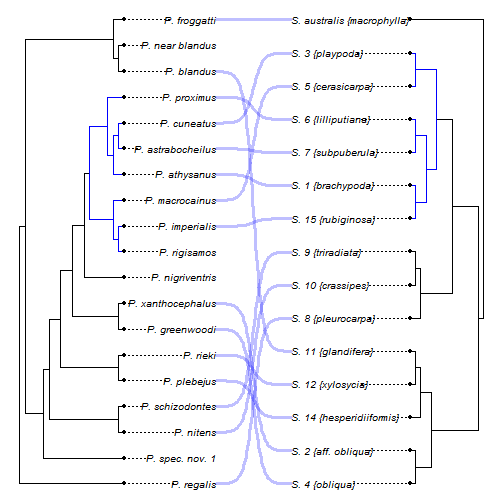``````## -- phytools contains a method for plotting a posterior sample of trees from
## -- Bayesian analysis. This is more or less equivalent to the stand-alone
## -- program densiTree. Here's a demo:

``````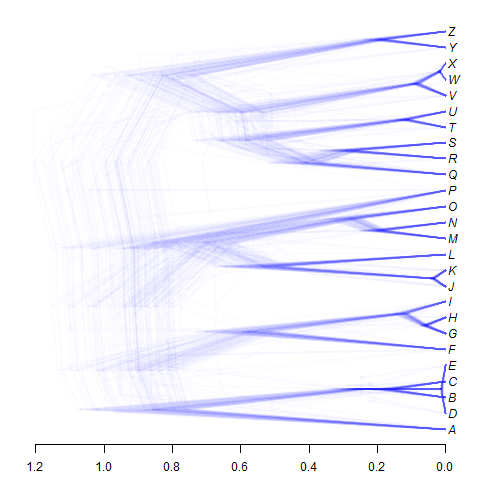``````## -- We can also just represent topological incongruence among trees:

densityTree(trees,use.edge.length=FALSE,type="phylogram",nodes="inner")
``````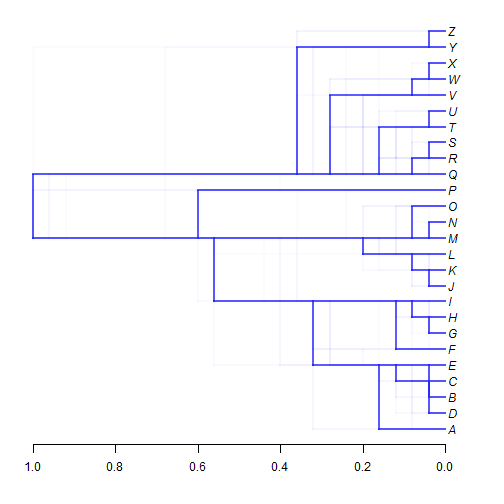``````## -- If we want to just compare two alternative time trees for the same set
## -- of taxa, we could try compare.chronograms. Here is a demo with simulated
## -- trees.

tree<-rtree(n=26,tip.label=LETTERS)
t1<-force.ultrametric(tree,"nnls")
tree\$edge.length<-runif(n=nrow(tree\$edge))
t2<-force.ultrametric(tree,"nnls")
compare.chronograms(t1,t2)
``````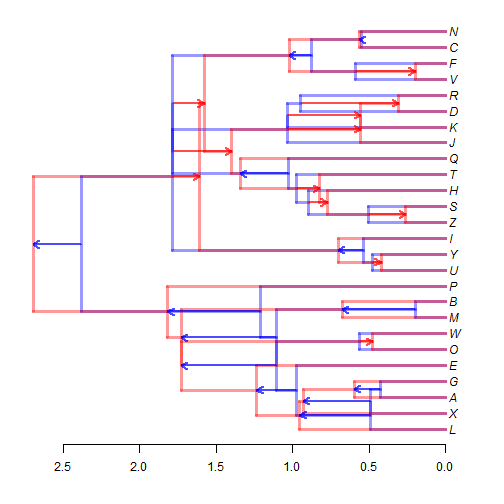``````## -- phytools has an interactive node labeling method. Here's a demo using a
## -- phylogeny of vertebrates (in non-interactive mode)

plotTree(vertebrates,xlim=c(-24,450))
labels<-c("Cartilaginous fish",
"Ray-finned fish",
"Lobe-finned fish",
"Anurans",
"Reptiles (& birds)",
"Birds",
"Mammals",
"Eutherians")
nodes<-labelnodes(text=labels,node=c(24,27,29,32,34,35,37,40),
shape="ellipse",cex=0.8,interactive=FALSE)
``````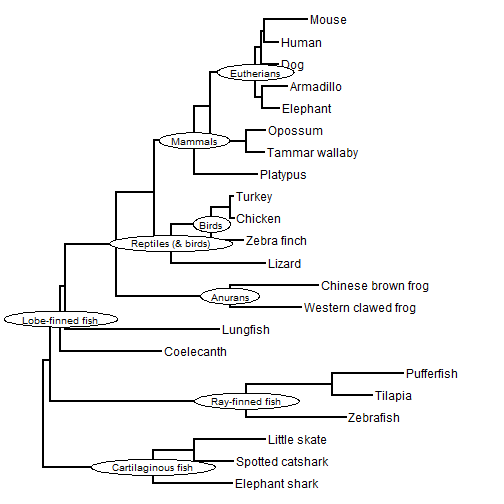``````## -- Another method in phytools allows us to easily label only a subset of tips
## -- in the tree. This can be handy for very large trees in which we are only
## -- interested in labeling a handful of taxa.

plot(anoletree,cols,xlim=c(0,9),ylim=c(-4,Ntip(anoletree)),ftype="off")
text<-sample(anoletree\$tip.label,20)
tips<-sapply(text,function(x,y) which(y==x),y=anoletree\$tip.label)
``````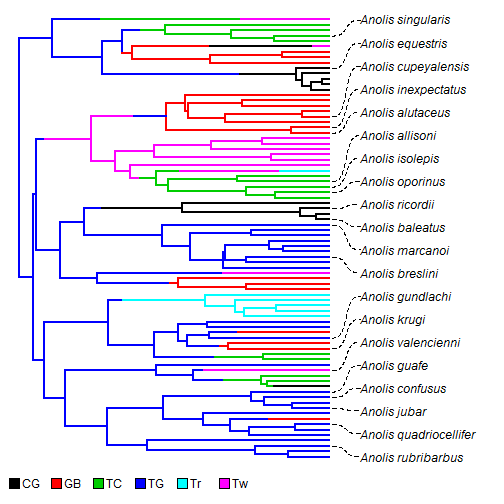``````## -- Here is a recent method for adding circular clade labels to a
## -- plotted fan style tree.

obj<-setMap(contMap(anole.tree,svl,plot=FALSE),invert=TRUE)
plot(obj,type="fan",ftype="off",lwd=c(2,6),outline=FALSE,xlim=c(-1.3,1.3),
legend=0.8,leg.txt="log(SVL)")
nodes<-c(105,117,122,135,165,169,68,172,176,183,184)